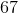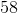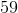# Common Core: 1st Grade Math : Understanding Place Value

## Example Questions

### Example Question #81 : Understanding Place Value

What digit is in the ones place?Explanation:

The ones place is always the first number on the right.

### Example Question #82 : Understanding Place Value

What digit is in the ones place?Explanation:

The ones place is always the first number on the right.

### Example Question #83 : Understanding Place Value

What digit is in the ones place?Explanation:

The ones place is always the first number on the right.

### Example Question #84 : Understanding Place Value

What digit is in the tens place?Explanation:

The tens place is always the second number from the right.

### Example Question #85 : Understanding Place Value

What digit is in the tens place?Explanation:

The tens place is always the second number from the right.

### Example Question #86 : Understanding Place Value

What digit is in the tens place?Explanation:

The tens place is always the second number from the right.

### Example Question #87 : Understanding Place Value

What digit is in the tens place?Explanation:

The tens place is always the second number from the right.

### Example Question #88 : Understanding Place Value

What digit is in the tens place?Explanation:

The tens place is always the second number from the right.

### Example Question #89 : Understanding Place Value

What digit is in the tens place?Explanation:

The tens place is always the second number from the right.

### Example Question #90 : Understanding Place Value

What digit is in the tens place?